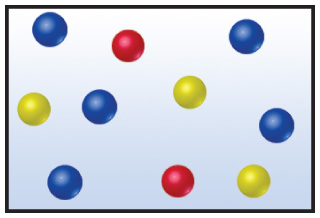# Problem: The drawing below represents a mixture of three different gases.If the total pressure of the mixture is 1.55 atm, calculate the partial pressure of the red gas.

###### FREE Expert Solution

Recall that the partial pressure of a gas (PGas) in a mixture is given by:

$\overline{){{\mathbf{P}}}_{{\mathbf{Gas}}}{\mathbf{=}}{{\mathbf{X}}}_{\mathbf{G}\mathbf{a}\mathbf{s}}{{\mathbf{P}}}_{{\mathbf{Total}}}}\phantom{\rule{0ex}{0ex}}$

where χGas = mole fraction of the gas and Ptotal = total pressure of the gas mixture

The mole fraction of the gas is then given by:

80% (491 ratings)###### Problem Details

The drawing below represents a mixture of three different gases.If the total pressure of the mixture is 1.55 atm, calculate the partial pressure of the red gas.